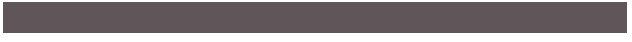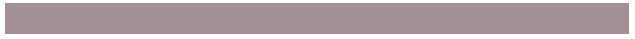Javascript | How to lighten a color by some percentage

Author - Webner
28.11.2017
|
|

We can lighten any color using a custom javascript function:

function shadeColor(color, percent) {
var R = parseInt(color.substring(1,3),16);
var G = parseInt(color.substring(3,5),16);
var B = parseInt(color.substring(5,7),16);

R = parseInt(R * (100 + percent) / 100);
G = parseInt(G * (100 + percent) / 100);
B = parseInt(B * (100 + percent) / 100);

R = (R<255)?R:255;
G = (G<255)?G:255;
B = (B<255)?B:255;

var RR = ((R.toString(16).length==1)?"0"+R.toString(16):R.toString(16));
var GG = ((G.toString(16).length==1)?"0"+G.toString(16):G.toString(16));
var BB = ((B.toString(16).length==1)?"0"+B.toString(16):B.toString(16));

return "#"+RR+GG+BB;
}

You need to pass a Hashcode (#605558 for example) of the color that you want to lighten as the first parameter in the function and the 2nd parameter is the percentage i.e. how much you want to lighten the color. The function will return the hashcode of lightened color.

For Example: